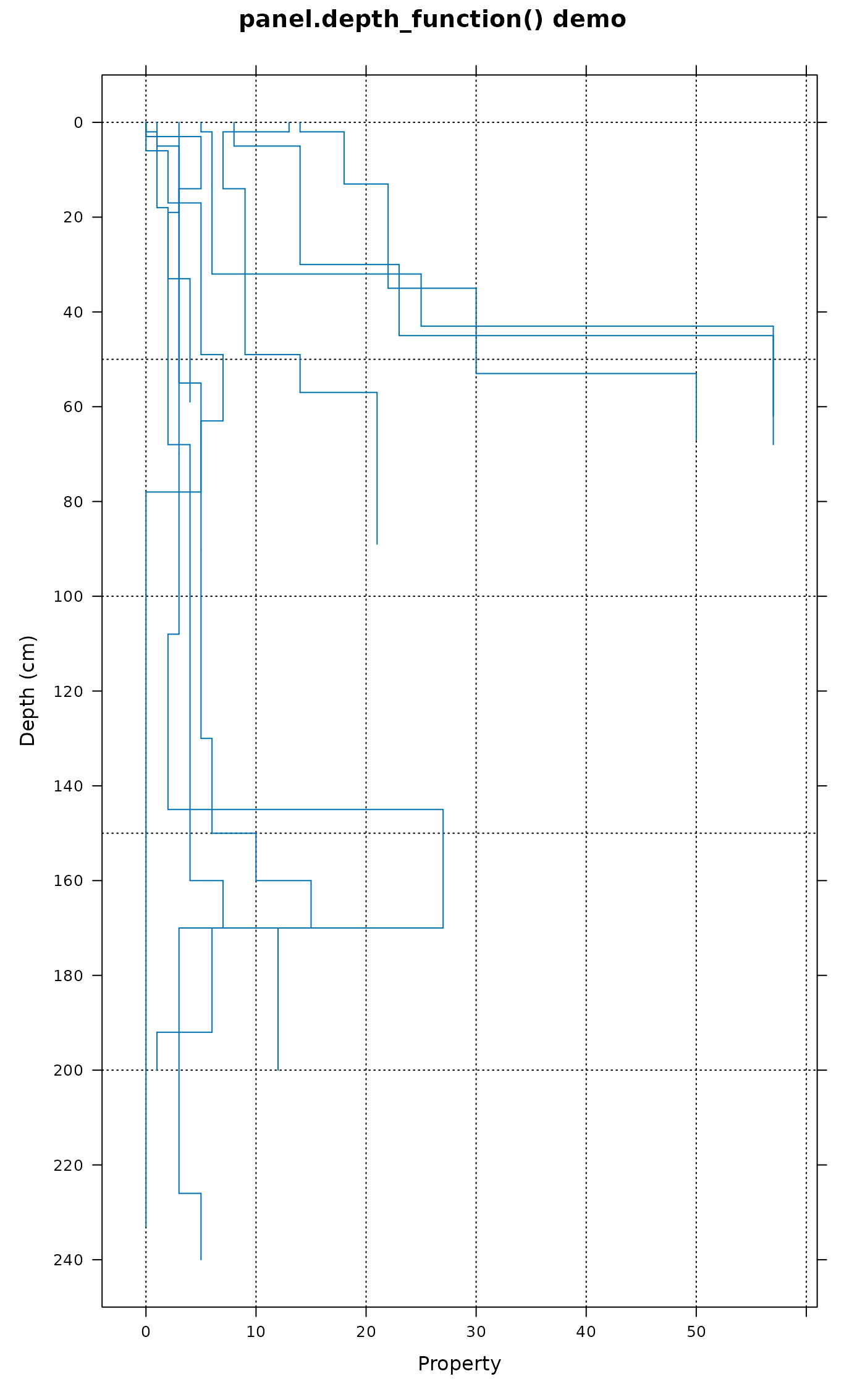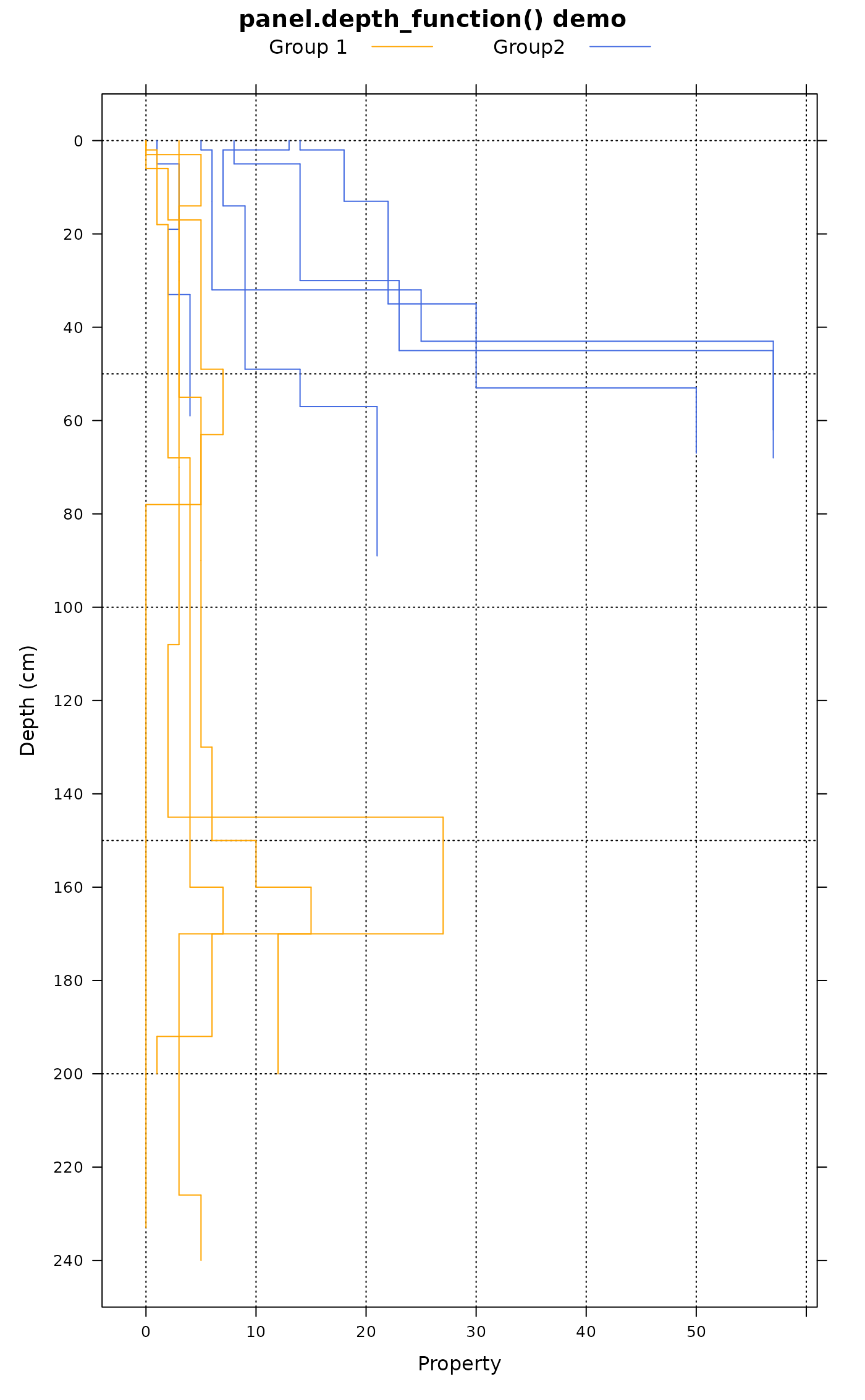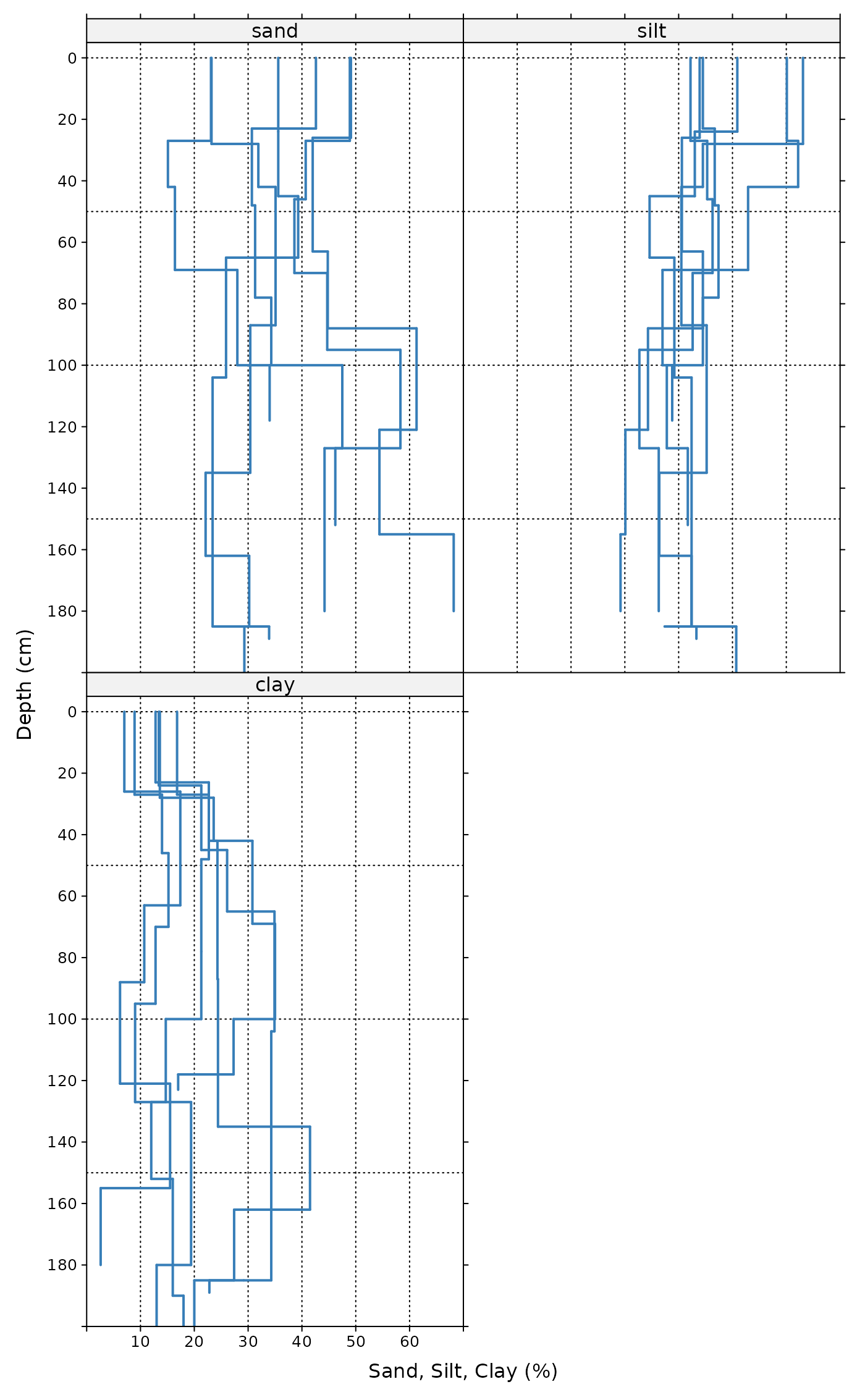Panel function for plotting grouped soil property data, along with upper and lower estimates of uncertainty.

This function can be used to replace panel.superpose when plotting depth function data. When requested, contributing fraction data are printed using colors the same color as corresponding depth function lines unless a single color value is given via cf.col.

This function is not able to apply transformations (typically log = 10) applied in the scales argument to xyplot to upper/lower bounds. These will have to be manually applied. See examples.

panel.depth_function(
x,
y,
id,
upper = NA,
lower = NA,
subscripts = NULL,
groups = NULL,
sync.colors = FALSE,
cf = NA,
cf.col = NA,
cf.interval = 20,
...
)

## Arguments

x

x values (generated by calling lattice function)

y

y values (generated by calling lattice function)

id

vector of id labels, same length as x and y--only required when plotting segments (see Details section)

upper

vector of upper confidence envelope values

lower

vector of lower confidence envelope values

subscripts

paneling indices (generated by calling lattice function)

groups

grouping data (generated by calling lattice function)

sync.colors

optionally sync the fill color within the region bounded by (lower--upper) with the line colors

cf

optionally annotate contributing fraction data at regular depth intervals see slab

cf.col

optional color for contributing fraction values, typically used to override the line color

cf.interval

number of depth units to space printed contributing fraction values

...

further arguments to lower-level lattice plotting functions, see below

sp1, slice, slab

D.E. Beaudette

## Examples


library(lattice)
data(sp1)

# 1. plotting mechanism for step-functions derived from soil profile data
xyplot(
cbind(top, bottom) ~ prop,
data = sp1,
id = sp1$id, panel = panel.depth_function, ylim = c(250, -10), scales = list(y = list(tick.number = 10)), xlab = 'Property', ylab = 'Depth (cm)', main = 'panel.depth_function() demo' )# 1.1 include groups argument to leverage lattice styling framework sp1$group <- factor(sp1$group, labels = c('Group 1', 'Group2')) xyplot( cbind(top, bottom) ~ prop, groups = group, data = sp1, id = sp1$id,
panel = panel.depth_function,
ylim = c(250, -10),
scales = list(y = list(tick.number = 10)),
xlab = 'Property',
ylab = 'Depth (cm)',
main = 'panel.depth_function() demo',
auto.key = list(
columns = 2,
points = FALSE,
lines = TRUE
),
par.settings = list(superpose.line = list(col = c(
'Orange', 'RoyalBlue'
)))
)# more complex examples, using step functions with grouped data
# better looking figures with less customization via tactile package
if(requireNamespace('tactile')) {

library(data.table)
library(lattice)
library(tactile)

# example data
data(sp6)

# a single profile
x <- sp6[1:5, ]

# wide -> long format
x.long <- melt(
data.table(x),
id.vars = c('id', 'top', 'bottom'),
measure.vars = c('sand', 'silt', 'clay')
)

# (optional) convert back to data.frame
x.long <- as.data.frame(x.long)

# three variables sharing a common axis
# factor levels set by melt()
xyplot(
cbind(top, bottom) ~ value | id,
groups = variable,
data = x.long,
id = x.long$id, ylim = c(200, -5), xlim = c(10, 60), scales = list(alternating = 1, y = list(tick.number = 10)), par.settings = tactile.theme(superpose.line = list(lwd = 2)), xlab = 'Sand, Silt, Clay (%)', ylab = 'Depth (cm)', panel = panel.depth_function, auto.key = list(columns = 3, lines = TRUE, points = FALSE), asp = 1.5 ) # all profiles x <- sp6 # wide -> long format x.long <- melt( data.table(x), id.vars = c('id', 'top', 'bottom'), measure.vars = c('sand', 'silt', 'clay') ) # (optional) convert back to data.frame x.long <- as.data.frame(x.long) # three variables sharing a common axis # factor levels set by melt() xyplot( cbind(top, bottom) ~ value | id, groups = variable, data = x.long, id = x.long$id,
ylim = c(200, -5), xlim = c(0, 70),
scales = list(alternating = 1, y = list(tick.number = 10)),
par.settings = tactile.theme(superpose.line = list(lwd = 2)),
xlab = 'Sand, Silt, Clay (%)',
ylab = 'Depth (cm)',
panel = panel.depth_function,
auto.key = list(columns = 3, lines = TRUE, points = FALSE),
as.table = TRUE
)

xyplot(
cbind(top, bottom) ~ value,
groups = variable,
data = x.long,
id = x.long$id, ylim = c(200, -5), xlim = c(0, 70), scales = list(alternating = 1, y = list(tick.number = 10)), par.settings = tactile.theme(superpose.line = list(lwd = 2)), xlab = 'Sand, Silt, Clay (%)', ylab = 'Depth (cm)', panel = panel.depth_function, auto.key = list(columns = 3, lines = TRUE, points = FALSE), as.table = TRUE ) xyplot( cbind(top, bottom) ~ value | variable, groups = variable, data = x.long, id = x.long$id,
ylim = c(200, -5), xlim = c(0, 70),
scales = list(alternating = 1, y = list(tick.number = 10)),
par.settings = tactile.theme(superpose.line = list(lwd = 2)),
xlab = 'Sand, Silt, Clay (%)',
ylab = 'Depth (cm)',
panel = panel.depth_function,
auto.key = list(columns = 3, lines = TRUE, points = FALSE),
as.table = TRUE
)

xyplot(
cbind(top, bottom) ~ value | variable,
data = x.long,
id = x.long\$id,
ylim = c(200, -5), xlim = c(0, 70),
scales = list(alternating = 1, y = list(tick.number = 10)),
par.settings = tactile.theme(superpose.line = list(lwd = 2)),
xlab = 'Sand, Silt, Clay (%)',
ylab = 'Depth (cm)',
panel = panel.depth_function,
auto.key = list(columns = 3, lines = TRUE, points = FALSE),
as.table = TRUE
)

}